## Example Questions

### Example Question #1 : How To Subtract Integers

On an airline flight of 250 people there is a choice of pasta, salad, or pizza for dinner. If 113 passengers choose pasta and 30% choose salad, how many passengers choose pizza?

62

53

100

75

62

Explanation:

30% of 250 is 75. Therefore, 250 – 75 – 113 = 62

### Example Question #2 : How To Subtract Integers

At a store, a t-shirt costs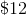. If Shane boughtt-shirts and shoes from the store for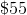, how much were the shoes?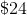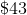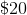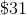Explanation:

If one shirt costs, then two shirts must cost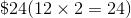. The difference of the total and this number is the cost of the the shoes.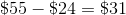### Example Question #3 : How To Subtract Integers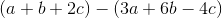is equivalent to which of the following?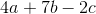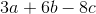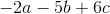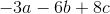Explanation:

To answer this question, we must remember how to distribute a negative when we are subtracting an entire expression.

To distribute a negative, you change the sign of everything within the expression being subtracted from positive to negative or negative to positive. You then add the resulting expression. So, for this data: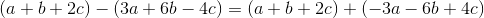We can then add the like expressions normally (combinewith,with, andwith). Therefore, for this data: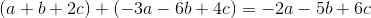Therefore, our answer is.